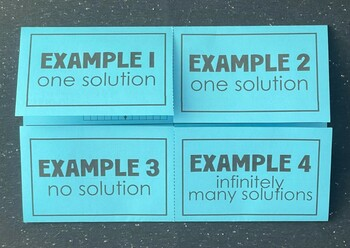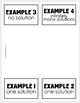# Graphing Linear Systems (Foldable)Subject
Resource Type
Format
Zip (589 KB|5 pages)
Standards
\$2.50
Also included in
1. PERFECT for interactive notebooks!All files include an answer key! You may also be interested in some of my mega foldable bundles: ★ The Ultimate Foldable & Activity Bundle for 5TH GRADE MATH★ The Ultimate Foldable & Activity Bundle for 6TH GRADE MATH★ The Ultimate Foldable & Activity Bu
\$16.00
\$30.00
Save \$14.00
• Product Description
• Standards
This foldable is organized into 4 examples in which students will need to graph linear equations in order to identify the solution to a system of linear equations. Many of the equations need to be manipulated into slope-intercept form, first. The first two examples have one distinct solution. Example 3 has no solution (parallel lines) and example 4 has infinitely many solutions (exact same line).

Now with a second option in which all equations are already written in slope-intercept form!

This is a GREAT item to add to you interactive notebook!

I used this to introduce linear systems to my 8th grade math classes.

An answer key is provided- this is especially helpful for absent students!

You may also be interested in some of my foldable bundles:

The Ultimate 7th GRADE MATH Foldable & Activity Bundle
The Ultimate PRE-ALGEBRA Foldable & Activity Bundle
The Ultimate PRE-ALGEBRA & ALGEBRA 1 Foldable Bundle
The Ultimate GEOMETRY Foldable Bundle
to see state-specific standards (only available in the US).
Solve systems of linear equations exactly and approximately (e.g., with graphs), focusing on pairs of linear equations in two variables.
Understand that solutions to a system of two linear equations in two variables correspond to points of intersection of their graphs, because points of intersection satisfy both equations simultaneously.
Total Pages
5 pages
Included
Teaching Duration
N/A
Report this Resource to TpT
Reported resources will be reviewed by our team. Report this resource to let us know if this resource violates TpT’s content guidelines.
• Ratings & Reviews
• Q & A

Teachers Pay Teachers is an online marketplace where teachers buy and sell original educational materials.